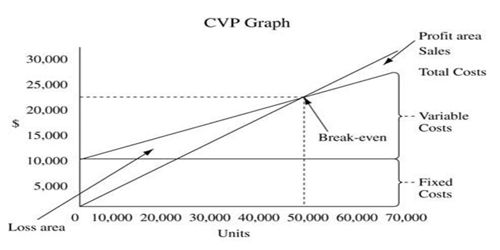Accounting

# Underlying Assumptions of CVP AnalysisCPV analysis is a powerful tool that helps managers understands the relationships of cost volume and profit. Cost volume profit (CVP) analysis is the relationship among cost, volume, and profit when output increases units cost of production decrease vice versa. It deals with how operating profit is affected by changes in variable costs, fixed costs, selling price per unit and the sales mix of two or more different products.

Assumptions Underlying CVP Analysis –

For any cost-volume-profit analysis to be valid, the following important assumptions must be reasonably satisfied within the relevant range.

• Selling price is constant; the price of the product or service will not change as volume changes.
• Costs are linear and can be accurately divided into variable and fixed elements. The variable element is constant per unit, and the fixed element is constant in total over the relevant range.
• In multi-product companies, the sales mix is constant.
• In manufacturing companies, inventories do not change. The number of units produced equals the number of units sold.

While some of these assertions may be violated in practice, the violations are usually not serious enough to call into question the basic validity of CVP analysis. For example, in most multi-product companies, the sales mix is constant enough so that the results of CVP analysts are reasonably valid./a